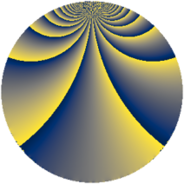Properties

 Label 240.2.blLevel $240$ Weight $2$ Character orbit 240.bl Rep. character $\chi_{240}(109,\cdot)$ Character field $\Q(\zeta_{4})$ Dimension $48$ Newform subspaces $1$ Sturm bound $96$ Trace bound $0$

Related objects

Defining parameters

 Level: $$N$$ $$=$$ $$240 = 2^{4} \cdot 3 \cdot 5$$ Weight: $$k$$ $$=$$ $$2$$ Character orbit: $$[\chi]$$ $$=$$ 240.bl (of order $$4$$ and degree $$2$$) Character conductor: $$\operatorname{cond}(\chi)$$ $$=$$ $$80$$ Character field: $$\Q(i)$$ Newform subspaces: $$1$$ Sturm bound: $$96$$ Trace bound: $$0$$

Dimensions

The following table gives the dimensions of various subspaces of $$M_{2}(240, [\chi])$$.

Total New Old
Modular forms 104 48 56
Cusp forms 88 48 40
Eisenstein series 16 0 16

Trace form

 $$48 q + O(q^{10})$$ $$48 q + 12 q^{10} - 16 q^{14} - 4 q^{16} + 8 q^{19} - 4 q^{24} - 40 q^{26} - 8 q^{30} - 48 q^{31} - 28 q^{34} + 24 q^{35} - 4 q^{36} - 16 q^{40} - 40 q^{44} - 4 q^{46} + 48 q^{49} - 32 q^{50} + 8 q^{51} - 4 q^{54} + 48 q^{56} - 32 q^{59} - 24 q^{60} + 16 q^{61} + 48 q^{64} + 16 q^{65} + 24 q^{66} - 16 q^{69} + 40 q^{74} - 16 q^{75} + 60 q^{76} - 96 q^{79} + 72 q^{80} - 48 q^{81} + 16 q^{86} + 8 q^{90} - 32 q^{91} + 44 q^{94} - 48 q^{95} - 40 q^{96} + O(q^{100})$$

Decomposition of $$S_{2}^{\mathrm{new}}(240, [\chi])$$ into newform subspaces

Label Dim. $$A$$ Field CM Traces $q$-expansion
$a_{2}$ $a_{3}$ $a_{5}$ $a_{7}$
240.2.bl.a $48$ $1.916$ None $$0$$ $$0$$ $$0$$ $$0$$

Decomposition of $$S_{2}^{\mathrm{old}}(240, [\chi])$$ into lower level spaces

$$S_{2}^{\mathrm{old}}(240, [\chi]) \cong$$ $$S_{2}^{\mathrm{new}}(80, [\chi])$$$$^{\oplus 2}$$